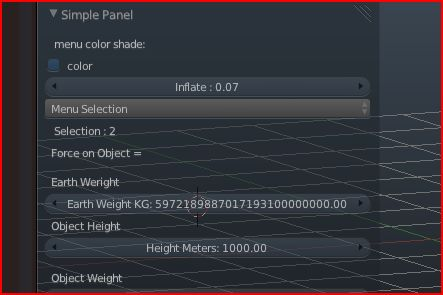field format in panel

i did a panel and when i add a button
i have a problem with the field size
there are too many numbers
how can you control the format of the number in the button ?
like can it be shown as a scientific format may be ?

thanks

These are the only options:

:arg precision: Number of digits of precision to display.

:arg subtype: Enumerator in [‘UNSIGNED’, ‘PERCENTAGE’, ‘FACTOR’, ‘ANGLE’, ‘TIME’, ‘DISTANCE’, ‘NONE’].

:arg unit: Enumerator in [‘NONE’, ‘LENGTH’, ‘AREA’, ‘VOLUME’, ‘ROTATION’, ‘TIME’, ‘VELOCITY’, ‘ACCELERATION’].

Afaik there’s no way to display as 1e-003 for instance, but controlling the visible number of floating point digits should be ok

ok but how to adjust number of digits ?
don’t se any format to do that !

right now i got something like 20 digits in UI
would like to show it like science
one number shows the earth mass which is huge in Kgi tought python was limited to 15 digits
how come we got more here
like 27 digits

thanks

you should read up on how computers represent floating point values

Makes no sense to me to use KG for world weight, use tons or whatever unit, e.g.

5974 Exatons

i know how to control this in a print or label with format
but not for a prop
also i tought bl was limited to 7 digits and python was at 15 digits

so not certain why we can see so many digits in prop button!
i can always change it to a megatons to have a smaller number

now i used Kg cause MKS use meter and KG

thanks

A precision to the 7th or whatever floating point digit doesn’t mean that there are 7 digits after the dot in decimal representation. Computers use the binary numbering system, and floating point numbers are actually fractions:

http://www.cprogramming.com/tutorial/floating_point/understanding_floating_point_representation.html

It doesn’t matter what unit system something uses, you can easily convert forth and back.

are you saying there is not way to change the format in the prop field?
or is there a way to show it as a sciencetific format

i can do it in a print with a format !
or in a lable field

thanks

you can’t turn a 1,000,000,000 into 1e+9 for a prop. You can however control the number of displayed floating point digits.

how to format it ?

where is the doc to control the format for float ?

i remember that in UI when you change the scale you might get something like 27 digits in the fields!
not certain why it does not convert to scientific format too!

thanks